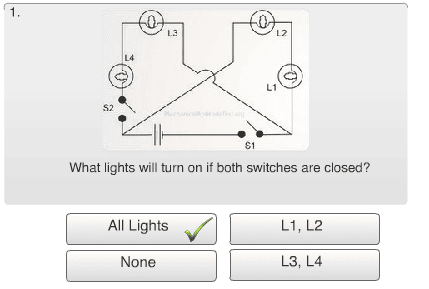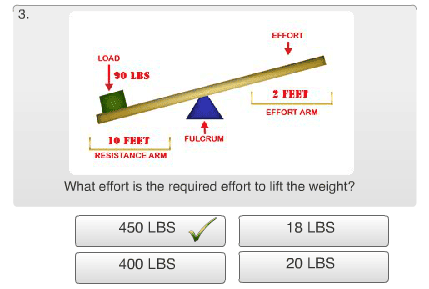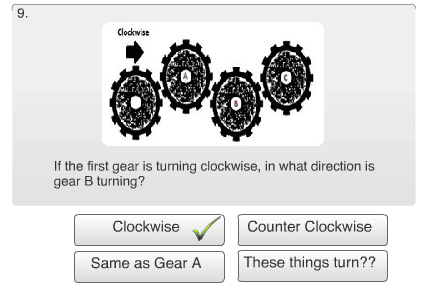• Home

# Free Mechanical Reasoning Test #1 – Review

So you have completed the FREE mechanical reasoning test. Now let’s have a look at the correct answers and go into detail on how to solve each question. So enough talk, let’s get right into it!

FREE Mechanical Reasoning Test – Question #1## Download Our Free Mechanical Aptitude eBook

Do you feel prepared for your next test?Explanation – Question #1

As we can see, the correct answer is, all lights. The reason being is in an electrical circuit, there needs to be a wire that sends out the power (hot wire) and a wire that allows current to return back to the source (Neutral). In this example, when you shut both S1 and S2, the current is able to reach all lights and the current is able to return to the source. Therefore, all lights are able to aluminate.

FREE Mechanical Reasoning Test – Question #2Explanation – Question #2

In order to answer this question correctly, you need to identify the piece of equipment. People tend to call it different things but a “Skills Saw” is a common name. A skill saw is used in mostly residential environments to help with carpentry. Therefore, once you have identified it’s name and purpose, it’s apparent that it is commonly used to cut plywood.

FREE Mechanical Reasoning Test – Question #3Explanation – Question #3

For a detailed explanation of this example, please visit our LEVERS SECTION. I will simply show the work in this review.

F=(90 x 10)/2
F=(900)/2
F= 450 LBS

FREE Mechanical Reasoning Test – Question #4Explanation – Question #4

In order to solve this problem you need to identify all the prongs. In this example, the bottom right is the hot, the bottom left is the neutral and the top one is the ground. A good way to identify the hot from the neutral is to have a look at a receptacle (plug). The blade that has a smaller opening is the hot. Therefore, in this example, the arrow is pointing to the ground.

FREE Mechanical Reasoning Test – Question #5Explanation – Question #5

When trying to figure out how to solve a pulley questions, it’s important to note that if the pulley is fixed (not moving), then the force required to pull the cord is equal to the weight. In the case that the pulley is not fixed, then the force is equal to half the weight. Therefore, in this example; considering that one pulley is fixed and one is moving, it would be 10/2=5.

FREE Mechanical Reasoning Test – Question #6Explanation – Question #6

To solve this one, you need to know how to identify a ballast. A ballast is a device intended to limit or increase the amount of current in an electric circuit. I would recommend that you familiarise yourself with common electrical components as they come up regularly in mechanical aptitude tests.

FREE Mechanical Reasoning Test – Question #7Explanation – Question #7

Once again, this is another example of a common mechanical aptitude question. This is a duck bill snip.

FREE Mechanical Reasoning Test – Question #8Explanation – Question #8

This is a plane question. I just recently decided to implement this type of question here at mechanicalaptitudetest.org because of multiple emails I was receiving about it. I was informed that this was also a common question on a mechanical aptitude test. The formula is as follows:

Effort X Length of plane = Resistance X Height to be raised
Unknown X 30 ft = 150 lb X 12 ft
X= 150lb x 12ft / 30ft
X= 1800ft-lb / 30
X= 60 lb of effort

FREE Mechanical Reasoning Test – Question #9Explanation – Question #9

It’s important to note that when you are given gears that are connected together; they are always turn in the opposite direction from each other. So in this example, if the first gear is turning clockwise, gear B is also running clockwise.

FREE Mechanical Reasoning Test – Question #10Explanation – Question #10

In this example, it asks you to turn on light L4. If have a close look you will notice that it requires both switches to be closed in order for the light to illuminate. Like I explained before, in order for a light to turn on, you require current to get to your light and current to return back to the source.

[ RETURN HOME ]